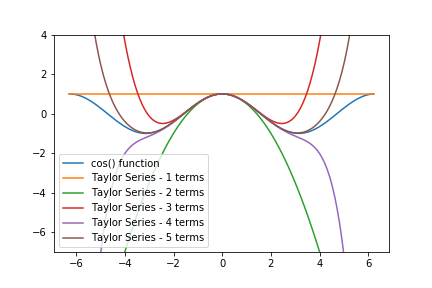## Exponents and Logs with PythonPython can be used just like a pocket calculator. In addition to addition, subtraction, multiplication and division, you can calculate exponents and logarithms with Python. Exponent and logarithm functions are imported from the math module which is part of the Python Standard Library. This means all the functions in the …

## How to do Trig with PythonPython can be used to complete trigonometric calculations. You can calculate sine, cosine, and tangent as well as use other trig functions using Python's math module, which is part of the Python Standard Library and comes included with all Python installations. (This post is adapted from my book Problem Solving …

## How to do Math with PythonPython can be used like a calculator to do math. Operations like addition, subtraction, multiplication, and division can be done with Python. In this post, you'll learn how to do math with Python.

## Arithmetic¶

Python can be used as a calculator to make arithmetic calculations.

Try the following commands in …

## Taylor Series in PythonIn this post, we will review how to create a Taylor Series with Python and for loops. Then we will refactor the Taylor Series into functions and compare the output of our Taylor Series functions to functions from Python's Standard Library.

A Taylor Series is an infinite series of mathematical …